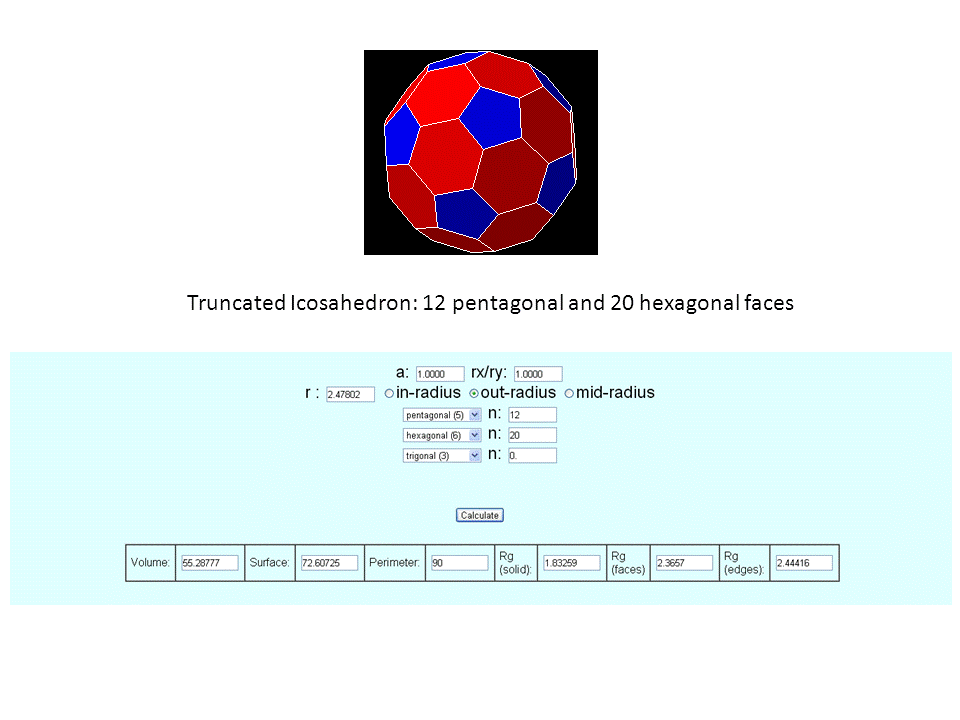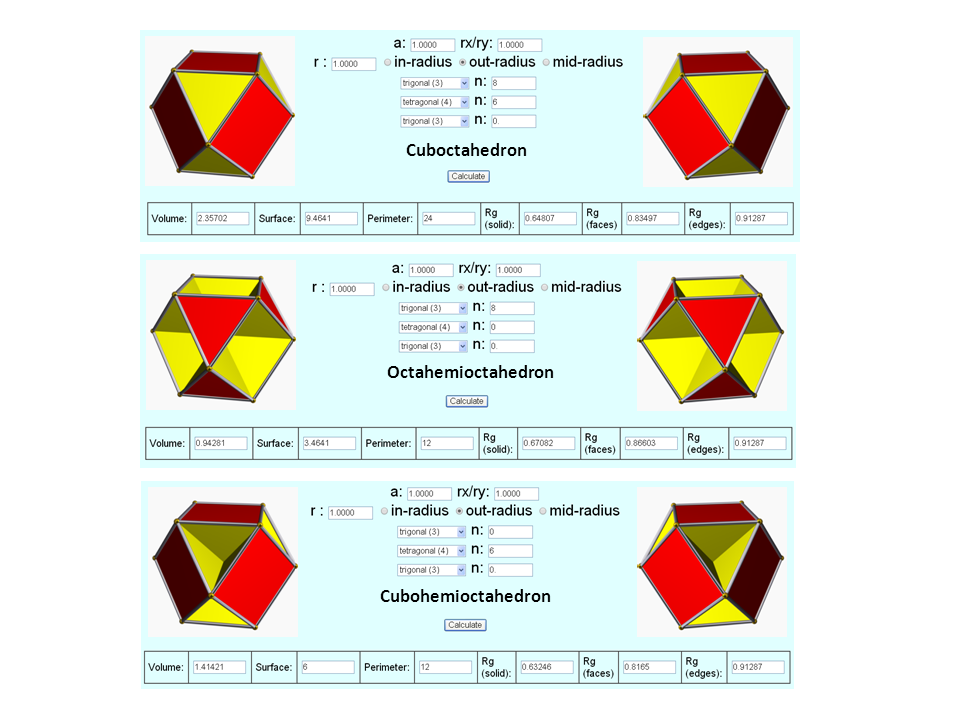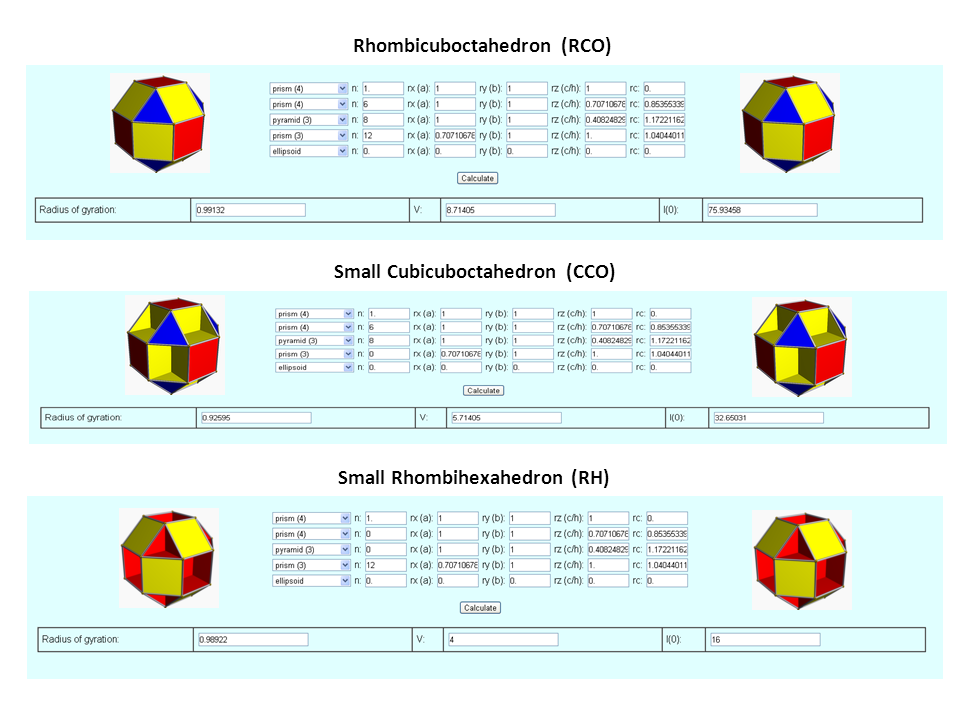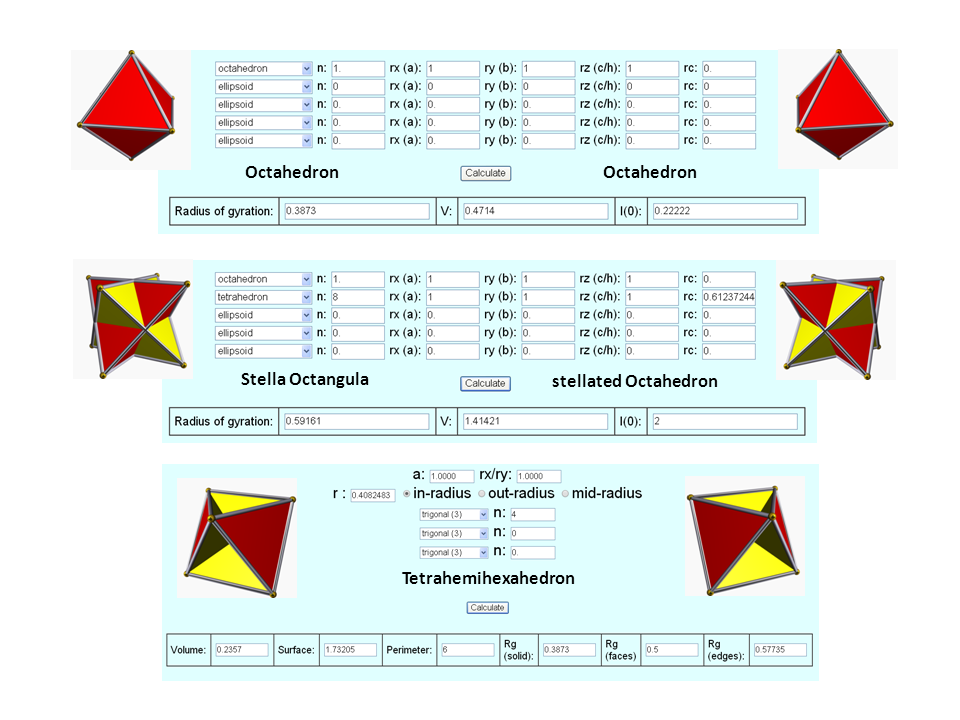## Calculation of the Radius of Gyration (Rg) of the Truncated Icosahedron, Cuboctahedron, Rhombicuboctahedron, Octahedron and of their variations.

As an example, the Radius of Gyration (Rg) of a Truncated Icosahedron (the shape of the C60-molecule) is being calculated. The polyhedral shape consists of 20 hexagons and 12 pentagons (60 vertices, 32 faces, 90 edges) with an unilateral edge-length a (here we take unit length = 1). All parameters for the calculation of Rg, such as out-, mid- and in-sphere radii (ro, rm, ri), respectively, can be found at Wolfram MathWorld .

The parameters for the solid shape are entered as input into the Rg-calculator as shown below:Parameter (Truncated Icosahedron) Value Volume 55.288 Surface 72.607 Perimeter 90.000 out-sphere radius ro 2.478 mid-sphere radius rm 2.427 in-sphere radius ri (pentagons) 2.327 in-sphere radius ri (hexagons) 2.267 Rg of solid 1.833 Rg of faces 2.366 Rg of edges 2.444 Rg of vertices ( = ro) 2.478

Table 1. Shape parameters and calculated values for Rg (rounded) of the regular truncated icosahedron (C60) of unit length a=1 ( = edge length). Rg scales linearly with the edge-length a. Units are arbitrary.As another example, the Radius of Gyration (Rg) of the Cuboctahedron (CO) and of two of its variations (Octahemioctahedron (OH) and Cubohemioctahedron (CH)) are being calculated. The polyhedral shape consists of 8 trigons and 6 tetragons (12 vertices, 14 faces, 24 edges) with an unilateral edge-length a (here we take unit length = 1). All parameters for the calculation of Rg, such as out-, mid- and in-sphere radii (ro, rm, ri), respectively, can be found at Wolfram MathWorld .

The parameters for the solid shape are entered as input into the Rg-calculator as shown below:Parameter (Cuboctahedron) Value Volume 2.357 Surface 9.464 Perimeter 24.000 out-sphere radius ro 1.000 mid-sphere radius rm 0.866 in-sphere radius ri (trigons) 0.816 in-sphere radius ri (tetragons) 0.707 Rg (CO) 0.648 Rg (OH) 0.671 Rg (CH) 0.632

Table 2. Shape parameters and calculated values for Rg (rounded) of the Cuboctahedron and of two variations with unit length a=1 ( = edge length). Rg scales linearly with the edge-length a. Units are arbitrary.Next, the Radius of Gyration (Rg) of the Small Rhombicuboctahedron (RCO) and of two of its variations (Small Cubicuboctahedron (CCO) and Small Rhombihexahedron (RH)) are being calculated. The polyhedral shape consists of 8 trigons and 18 tetragons (24 vertices, 26 faces, 48 edges) with an unilateral edge-length a (here we take unit length = 1). All parameters for the calculation of Rg, such as out-, mid- and in-sphere radii (ro, rm, ri), respectively, can be found at Wolfram MathWorld .

The parameters for the solid shape are entered as input into the Rg-calculator as shown below:Parameter (Small Rhombicuboctahedron) Value Volume 8.714 Surface 21.464 Perimeter 48.000 out-sphere radius ro 1.399 mid-sphere radius rm 1.307 in-sphere radius ri (trigons) 1.274 in-sphere radius ri (tetragons) 1.207 Rg (RCO) 0.991 Rg (CCO) 0.926 Rg (RH) 0.989

Table 3. Shape parameters and calculated values for Rg (rounded) of the Samll Rhombicuboctahedron and of two variations with unit length a=1 ( = edge length). Rg scales linearly with the edge-length a. Units are arbitrary.Last, the Radius of Gyration (Rg) of two variations of the Octahedron (Stella Octangula (SO) and Tetrahemihexahedron (THH)) are being calculated. The polyhedral shape (octahedron) consists of 8 trigons (6 vertices, 8 faces, 12 edges) with an unilateral edge-length a (here we take unit length = 1). In case of the Stalla Octangula all eight faces are stellated with tetrahedra of edge lengths equal to a, in case of the Tetrahemihexahedron four faces are removed (4 of the 8 trigonal pyramids are 'cut out'). In both cases the position of the centroid remains the same.

The parameters for the solid shape are entered as input into the Rg-calculator (and also here) as shown below:Parameter (Octahedron) Value Volume 0.471 Surface 3.464 Perimeter 12.000 out-sphere radius ro 0.707 mid-sphere radius rm 0.500 in-sphere radius ri (trigons) 0.408 Rg (O) 0.387 Rg (SO) 0.592 Rg (THH) 0.387

Table 3. Shape parameters and calculated values for Rg (rounded) of the Octahedron and of two variations with unit length a=1 ( = edge length). Note, Rg of the THH is the same as for the Octahedron, no matter how many trigonal pyramids are removed from the octagon (provided the location of the centroid remains the same). Rg scales linearly with the edge-length a. Units are arbitrary.Author: M.Kriechbaum, TU-Graz (2013), e-mail: manfred.kriechbaum@tugraz.at